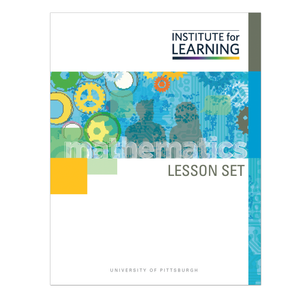# Writing and Comparing Decimal Numbers (Grade 4)

Regular price \$6.25 \$0.00 Unit price per

This lesson set develops understanding of the relationship between fractions and decimals, decimal number notation and addition, as well as comparison of decimal numbers. Students will:

• express a fraction with a denominator of 10 as an equivalent fraction with a denominator of 100 and write fractions with denominators of 10 and 100 with decimal notation;
• combine tenths and hundredths;
• compare two decimals to hundredths and justify the comparisons using visual models; and
• compare decimals and express their relationship using the symbols, >, <, or = and explain that comparisons are valid only when the two decimals refer to the same whole.

Standards: 4.NF.C.5, 4.NF.C.6, 4.NF.C.7. 4.NF.B.3